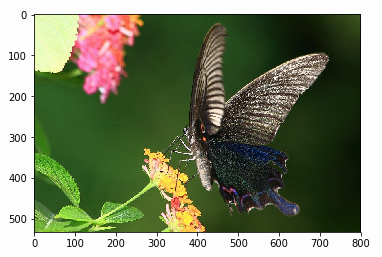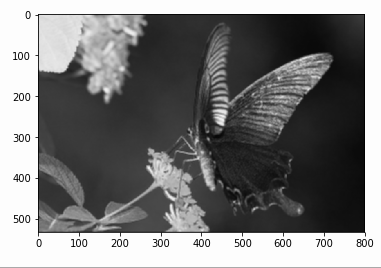## 2019/1/3

### CNN with TensorFlow

24 CNN with TensorFlow

🔶 CNN的重要classes

► tf.nn.conv2d

tf.nn.conv2d(
input,
filter,
strides,
use_cudnn_on_gpu=True,
data_format='NHWC',
dilations=[1, 1, 1, 1],
name=None
)
• input：指要做摺積的圖像輸入，要求是一個4-D Tensor，維度的次序是依data_format 參數的值而定，預設是 'NHWC'，指 [batch, height, width, channels]，N 是指，batch是要處理圖像的批量, H 是 height是指圖片高度, W 是 width是指圖片寬度,  C 是channels是指圖像通道數，亦即色彩空間的維度，這是一個4維的 Tensor，要求類型為half, bfloat16, float32, float64.。
• filter：相當於CNN中的摺積核，要求也是一個4-D Tensor，filter_height 指核的高度, filter_width 指核的寬度, in_channels 指輸入的通道數, out_channels 指輸出的通道數要求類型與參數input相同。
• strides：摺積時在圖像每一維的步長，這是一個一維的向量，長度4，對應到input 變數的每一維，各維次序依 data_format 設定，如用預設時  [1,2,2,1]是指每次高度及寬度都滑動兩個像素。第一個1指對應到批量，最後的1對應到in-channel，但我文獻裡找不到說明要如何在批量裡的不同圖像滑動，一般設成1，指在同一張圖像 。所以 strides  參數一般都是設成 [1,x,x,1]，x指想要在高及寬滑動的步長。
• use_cudnn_on_gpu: bool 類型，是否使用cudnn加速，預設為True
• data_format: 預設是 'NHWC'，也可改成 "NCHW"，這樣資料維度的次序變成[batch, ,channels, height, width]
• dilations: 是指膨脹，也就是核對應的接受場膨脹. 預設為 [1, 1, 1, 1]. 為長度為4 的 1-D 定義對每一輸入維度的膨脹係數。如果設 $k > 1$, 則在該維中，在每一個核元素間將會有$k-1$ cells (像素) 會被跳過，維度的次序依 data_format 參數設定。此設定在 batch 和 depth (channel) 的維度必需設為1.
• name: 對此操作取名，會顯示 於 graph 中 (選用)
• 結果返回一個tensor，這就是輸出的feature map
► tf.layers.conv2d
tf.layers.conv2d(
inputs,
filters,
kernel_size,
strides=(1, 1),
data_format='channels_last',
dilation_rate=(1, 1),
activation=None,
use_bias=True,
kernel_initializer=None,
bias_initializer=tf.zeros_initializer(),
kernel_regularizer=None,
bias_regularizer=None,
activity_regularizer=None,
kernel_constraint=None,
bias_constraint=None,
trainable=True,
name=None,
reuse=None
)


• inputs: Tensor 輸入。
• filters: 整數, 輸出空間的維度 (亦即摺積濾波器（核）的數目。
• kernel_size: 一個整數（方形）或是一個含兩整數的 tuple/list （矩形），指定2-D摺積的高度和寬度。 可以是一個整數指定所有空間維度相同值。
• padding: "valid" or "same" 兩者之一(大小寫均可)
• data_format: 一字串, channels_last (預設) 或 channels_first 兩者之一。指輸入各維的次序， channels_last 相當於輸入tensor的外形為 (batch, height, width, channels) 而channels_first 相當於輸入tensor的外形為 (batch, channels, height, width).
• dilation_rate: 一個整數或是一個含兩整數的 tuple/list,指定膨脹摺積的膨脹率 可以是一個整數指定所有空間維度相同值。目前指定任何膨脹率$!= 1$ 和指定stride $!= 1$ 並不相容。
• activation: 作動函數，設為 None 是線性作動。
• use_bias: 布林值, 指出此層是否使用偏置。
• kernel_initializer: 摺積核的初始器。
• bias_initializer: 偏置向量初始器，如果設成 None 將使用預設初始器。
• kernel_regularizer: 摺積核的最佳調節器。
• bias_regularizer: 偏置向量的最佳調節器。
• activity_regularizer: 輸出的最佳調節函數。
• kernel_constraint: 為一選用的投影函數，在由佳化器更新後作用到摺積核 (例如用於實現對層權重的範數限制或或數值限制). 此函數需以未投影變數為輸入，並以傳回投影變數(外形必需相同)。當執行非同步分散式訓時使用限制並不安全。
• trainable: 布林值, 如果設為 True 也會加變數到計算圖萃集 (graph collection) 的 GraphKeys.TRAINABLE_VARIABLES 。
• name: 一字串，此層的名稱。
• reuse: 布林值,是否要以相同的名稱重用先前某一層的權重。
► tf.nn.max_pool

tf.nn.max_pool(
value,
ksize,
strides,
data_format='NHWC',
name=None
)

• value:  格式依 data_format 參數的一個 4-D Tensor
• ksize: 4個整數的list. 指定對輸入tensor 每一維視窗尺度的整數。
• strides: 4個整數的list. 指定對輸入tensor 在每一維視每次滑 動步長。
• padding: 為 'VALID' or 'SAME' 字串
• data_format: A string. 'NHWC', 'NCHW'，且 'NCHW_VECT_C' 也有支援。
• name: 操作的名稱，為選用。.
► tf.layers.max_pooling2d

tf.layers.max_pooling2d
tf.layers.max_pooling2d(
inputs,
pool_size,
strides,
data_format='channels_last',
name=None
)

• inputs: 輸入的tensor，秩必需是4。
• pool_size: 池尺度，為一整數或是二個整數的 tuple/list 指定pool_height 和pool_width， 這指定出池化的框窗，一個整是就指正方形的框窗。
• strides: 歩長，為一整數或是二個整數的 tuple/list , 指定池化操作的步長， 一個整數是指在空間維度裡是相同步長。
• padding: 充填，為 'valid' or 'same'. 字串，大小寫都可。
• data_format: 有支援 channels_last (預設 和 channels_first 。 channels_last 相當於輸入是 (batch, height, width, channels) 而 channels_first 相當於輸入是 (batch, channels, height, width) 。（看來layers模組棄用NHWC 和NCHW）
• name: 此層的名稱。
► tf.nn.avg_pool

tf.nn.avg_pool(
value,
ksize,
strides,
data_format='NHWC',
name=None
)

• value:  外形為 [batch, height, width, channels] 的 4-D Tensor，資料形態為 float32, float64, qint8, quint8, or qint32.
• ksize: 4 個整數的 list. 在輸入tensor 各維上視窗的尺度。
• strides:  4 個整數的 list. 在輸入tensor 各維上滑動步長的大小。
• padding: 為 'VALID' 或r 'SAME' 字串。
• data_format: 字串. 可以是'NHWC' 或  'NCHW'。
• name: 此操作選用的名稱。
► tf.nn.pool

tf.nn.pool(
input,
window_shape,
pooling_type,
dilation_rate=None,
strides=None,
name=None,
data_format=None
)

• input: 秩為 N+2 的tensor, 如果data_format 不是以  "NC" 開頭 (預設) 則其外形為[batch_size] + input_spatial_shape + [num_channels]， 如果data_format 以  "NC" 開頭 ，則外形為 [batch_size, num_channels] + input_spatial_shape. 僅對空間維度施行池化。
• window_shape: $N$ 個整數序列 ，$N\geq 1$。此值就是空間維度的大小。
• pooling_type: 指定池化操作的種類，必需是 "AVG" 或 "MAX"。
• dilation_rate: 選用之膨脹率，為N整數的list.，預設為 *N. 如果有任何維度的 dilation_rate 大於1, 則所有 strides 必需1。
• strides: 選用之步長，為N整數的list.. 預設為 *N. 如果有任何維度的strides 大於1, 則所有  dilation_rate必需1。
• data_format: 為一字串或None. 指定輸入和輸出中通道是否為最後的維度 (預設值或是 data_format 不是以 "NC"開頭), 或者是位於第二維 (在 data_format 以 "NC"開頭的情況). 對 N=1, 此值是 "NWC" 預設) 和 "NCW". 對 N=2, 有效的設定為 "NHWC" (預設) 和 "NCHW". 對 N=3, 此值應為 "NDHWC" (預設) 和 "NCDHW"，其中D是指空間的第3維。
• 此函數傳回秩為$N+2$的tensor，其外形和data_format, padding, strides等參數有關。
► tf.layers.dense

tf.layers.dense(
inputs,
units,
activation=None,
use_bias=True,
kernel_initializer=None,
bias_initializer=tf.zeros_initializer(),
kernel_regularizer=None,
bias_regularizer=None,
activity_regularizer=None,
kernel_constraint=None,
bias_constraint=None,
trainable=True,
name=None,
reuse=None
)

• inputs: Tensor 輸入。
• units: Integer or Long, 輸出空間的維度
• activation: 作動函數（可叫用的），設為 None 是線性作動。
• use_bias: 布林值, 指出此層是否使用偏置。
• kernel_initializer: 摺積核的初始值。
• bias_initializer: 偏置向量初始值。
• kernel_regularizer: 摺積核的最佳調節器。
• bias_regularizer: 偏置向量的最佳調節器。
• activity_regularizer: 輸出的最佳調節函數。
• kernel_constraint: 為一選用的投影函數，在由佳化器更新後作用到摺積核 (例如用於實現對層權重的範數限制或或數值限制). 此函數需以未投影變數為輸入，並以傳回投影變數(外形必需相同)。當執行非同步分散式訓時使用限制並不安全。
• bias_constraint: 為一選用的投影函數，在由佳化器更新後作用到偏置
• trainable: 布林值, 如果設為 True 也會加變數到計算圖收集 (graph collection) 的 GraphKeys.TRAINABLE_VARIABLES 。
• name: 一字串，此層的名稱。
• reuse: 布林值,是否要以相同的名稱重用先前某一層的權重。
► tf.contrib.layers.fully_connected
fully_connected 層產生一個叫weights的變數，表示全連接的權重矩陣, 此矩陣和 inputs 相乘產生一個隱藏單元的 Tensor，如果有提供 normalizer_fn (如 batch_norm),那就會應用上，如果 normalizer_fn 設成 None， 且有提供 biases_initializer，那麼會產生 一biases變數並且加入隱藏單元最後，如果 activation_fn 不是 None, 也會應用到隱藏單元。此函數的參數如下
tf.contrib.layers.fully_connected(
inputs,
num_outputs,
activation_fn=tf.nn.relu,
normalizer_fn=None,
normalizer_params=None,
weights_initializer=initializers.xavier_initializer(),
weights_regularizer=None,
biases_initializer=tf.zeros_initializer(),
biases_regularizer=None,
reuse=None,
variables_collections=None,
outputs_collections=None,
trainable=True,
scope=None
)

• inputs: 一秩至少2的tensor 且最後維為靜態變數，亦即 [batch_size, depth], [None, None, None, channels].
• num_outputs: Integer or Long, 輸出單元的數目 。
• activation_fn: 作動函數. 預設值是 ReLU 函數. 明確地設成  None 可跳過並維持線性作動 。
• normalizer_fn:為用以替換 biases 的正規化函數. 如果有提供 normalizer_fn 則 biases_initializer 和 biases_regularizer 會被忽略， biases 路既不產生也不加入。預設值是設 None 為沒有 normalizer function。
• normalizer_params: 正規化函數的參數。
• weights_initializer: 權重的啟始值。
• weights_regularizer: 選用的權重調節器 。
• biases_initializer: biases 的初始值，假設沒有略過 biases。
• biases_regularizer: 選用的 biases 調節器。
• reuse: 是否此層和其變數要被重用. 若要能重用 scope 必需給定。
• variables_collections: 選用的所有變數的總集的 list或是含有每一變數一總集的不同list 的字典 。
• outputs_collections: 要加到輸出的收集。
• .trainable:如果設為 True 也會加變數到計算圖收集的 GraphKeys.TRAINABLE_VARIABLES 。
• scope: 選用的範圍，設定變數的有效範圍 。
► tf.nn.dropout

tf.nn.dropout(
x,
keep_prob,
noise_shape=None,
seed=None,
name=None
)

• x: 一個浮點數的 tensor.
• keep_prob: 一個類型(type) 和 x 一樣的純量 Tensor ，設定每一元素被保持住的機率。
• noise_shape: 為一 type 為int32的 1-D Tensor 表示隨機產生的keep/drop 旗標的外形。
• seed:  為一Python 整數，用以產隨機的種子。
• name: 選用的此操作的名稱。
► tf.nn.dropout  展平輸入的 tensor，但保持批量軸 (axis 0)
tf.layers.flatten(
inputs,
name=None
)

• inputs: Tensor 輸入
• name: 此層的名稱 (字串).
🔶 conv2d 操作實例

$L$: 樣本圖的長度
$K$: 核的長度
$P$: 在此維兩端填0的數目
$S$: 步長

import numpy as np
import tensorflow as tf
sess = tf.InteractiveSession()
img = tf.constant([1,2,3,4,5,6,7,8,9],dtype=tf.float32)
ker = tf.constant([1,2,3,4],dtype=tf.float32)
img = tf.reshape(img,[3,3])
ker = tf.reshape(ker,[2,2])
print(img.eval())
print(ker.eval())
img = tf.reshape(img,[1,3,3,1])
ker = tf.reshape(ker,[2,2,1,1])
activate_mp1 = tf.nn.conv2d(img,ker,strides = [1,1,1,1],padding ='VALID')
activate_mp2 = tf.nn.conv2d(img,ker,strides = [1,1,1,1],padding ='SAME')
print(activate_mp1.eval().shape)
activate_mp1 = tf.squeeze(activate_mp1)
activate_mp2 = tf.squeeze(activate_mp2)
print(activate_mp1.eval())
print(activate_mp2.eval())
sess.close()

[[1. 2. 3.]
[4. 5. 6.]
[7. 8. 9.]]
[[1. 2.]
[3. 4.]]
(1, 2, 2, 1)
[[37. 47.]
[67. 77.]]
[[37. 47. 21.]
[67. 77. 33.]
[23. 26.  9.]]


from PIL import Image
import matplotlib.pyplot as plt
import numpy as np
import tensorflow as tf
img = Image.open('butterfly.jpg')
plt.imshow(img)
pix = np.array(img)
print(pix.shape)
pix = np.expand_dims(pix, axis=0)
print(pix.shape)
#(533, 800, 3)
#(1, 533, 800, 3)輸入的圖片

sess = tf.InteractiveSession()
filter = np.full([5,5,3],1/75)
filter = np.expand_dims(filter, axis=3)
print(filter.shape)
img_tf = tf.constant(pix,dtype=tf.float32)
ker_tf = tf.constant(filter,dtype=tf.float32)
activate_mp3 = tf.nn.conv2d(img_tf,ker_tf,strides = [1,1,1,1],padding ='SAME')
result = activate_mp3.eval()
print(result.shape)
result = tf.squeeze(result)
result = result.eval()
sess.close()
img3 = Image.fromarray(result)
plt.imshow(img3)
#(5, 5, 3, 1)
# (1, 533, 800, 1)得到的輸出

https://www.tensorflow.org/tutorials/